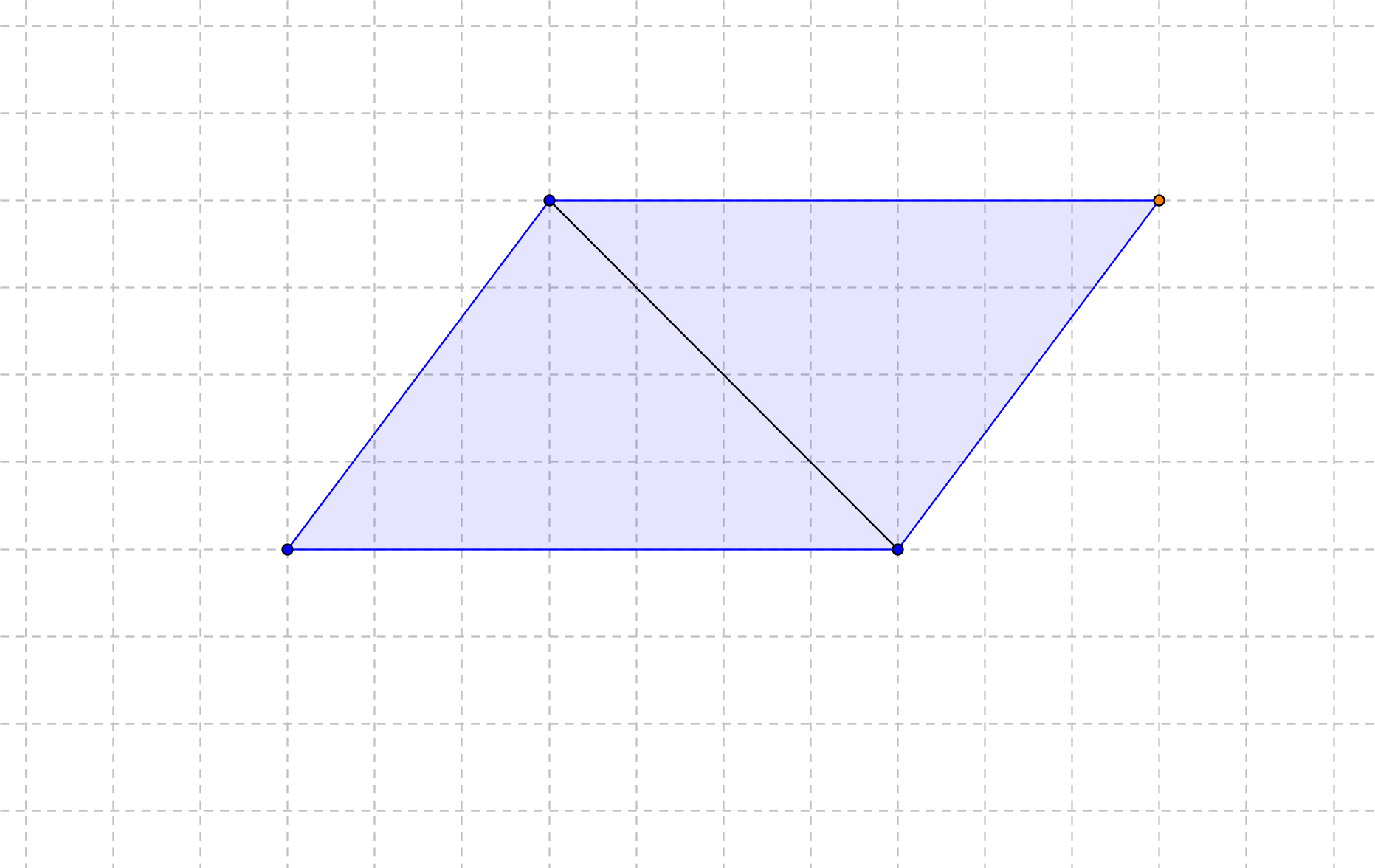# GEOM 1 | Lesson 1 | Explore (Area of Triangles and Trapezoids)# Area of Triangles and Trapezoids

Area of a Triangle

Next, take a parallelogram and cut the parallelogram into 2 equal pieces.

How will you determine the area of a triangle?Area of a Trapezoid:

Next, change the shape from a parallelogram to a trapezoid by cutting one of the triangle shaped pieces off the side of the third parallelogram.Now calculate the area of the patio by using areas of shapes you are familiar with.